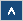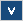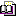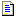Verilog Online HelpTable of ContentsBit-selectBlock StatementsBuilt-in PrimitivesCase StatementContinuous AssignmentsConversion FunctionsCommentsCompiler DirectivesConcatenationsConditional OperatorDelaysDisable StatementDisplay TasksEdge Sensitive PathExpression Bit LengthFile I/O FunctionsFunctionsIdentifiersIf StatementInteger ConstantsIntra-assignment Timing ControlsKeywordsLoop StatementsMemoriesmin:typ:max DelaysModule DeclarationModule InstantiationModule Path DeclarationModule Path PolarityNet Data TypesOperatorsParametersPart-selectPLA Modeling TasksProbabilistic Distribution FunctionsProcedural AssignmentsProcedural Continuous AssignmentsProcedural Timing ControlRange SpecificationReal ConstantsRegister Data TypesSimulation Control TasksSimulation Time FunctionsSpecify BlockState Dependent PathStochastic Analysis TasksStrengthsStringsStructured ProceduresTasksTimescale System TasksTiming Check TasksUDP DeclarationUDP InstantiationUDP State TableValue Change Dump (VCD) FileVectors

# Bit-select

## Simplified Syntax

vector_identifier[expression];

## Description

The bit-select can be used to access individual bits of vector net or register data types. The bits can be addressed by using an expression. If the expression value is out of bounds or it returns z or x values, then the value returned by the reference is x. If one or more bits of the address returned by the expression have an x or z value, then the address expression is x.

The bit-select can be applied to any net vectors, regs, integers, and time register data types. The bit-selection of a register declared as real or realtime is illegal.

## Examples

Example 1

reg [3:0] vect;
vect = 4'b0001;

If the value of address expression is 0 then returned value is 1 (vect = 1).

If the value of address expression is 3 then returned value is 0 (vect = 0).

If the value of address expression is 4 then returned value is x (vect = x).

If the value of address expression is x or z then returned value is x (vect[1'bx] = x).

Example 2

reg [0:3] vect;
vect = 4'b0001;

If the value of address expression is 3 then returned value is 1 (vect = 1).

If the value of address expression is 0 then returned value is 0 (vect = 0).

Example 3

reg [7:0] vect;
vect = 4;

Fills vect with the pattern 00000100 (MSB is bit 7, LSB is bit 0).

## Important Notes

• If the address expression is out of bounds or it returns an x or z value, then the returned value is x.

• The bit-select of real or realtime registers is illegal.